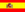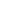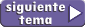home | más de cinemática | otros temas de Física | lecciones del maestro Ciruela | tonterías | @NO ME SALEN
EJERCICIOS RESUELTOS Y APUNTES DE FÍSICA Y BIOFÍSICA DEL CBCThe kinematic equations

If you are asked to write a kinematic equation, you have to write an expression wich includes x and t (x standing for distance and t for time). DO NOT REPLACE THEM for any numbers or sybmols or even money. Write x and t in any way you want but the expression MUST have a cross and a te; If  it doesn´t it might as well be anything but a kinematic equation.

Let me show you an example of the URM or MRU model:

x = xo + v ( tto )

x and t are variables, if those aren´t around, you are screwed; there´s no kinetic equation. The rest: xo, v and to are constants, numbers if you will, and if you are lucky you might get told the value of them. If you don´t know their value you might have to find it or decide it arbitrarily, but remember, They are not variables, they are constants! Onde they are settled that´s it, no more changes, their value will keep the same, like, I don´t know, here is an example::

x = 3 m – 4 m/s ( t  – 5 s )

That´s a kinetic equation, there´s no doubt about it, because you can see the x and the t right there. Besides I can guarantee you that xo = 3 m, v = – 4m/s y to = 5 s.

Another example:

x =  4 m/s ( t + 2 s )

In this example:  xo = 0 m, v = 4 m/s y to = – 2 s.

Perceive how you usually can distinguish what is a constant and what is a variable by just looking the subscript. The instant 0 - to- is unique and unrepeatable, that´s what we call a constant; However, t is the symbol of an infinite set, it represents any instant in time, it may represent to, ti, tA, the minute 34 or the hour 78.

A kinetic equation is an expresión capable of telling you where a movil is in any moment you want to know about, it is a warehouse full of data on kinematics that keeps infinite cases of time-space pairs. If you want to know about a movement and you have the kinetic equatio, that´s all, there are no more secrets. Neat.

Here I write another kinetic equation, but this one is a little bit different than the last kind of kinetic equation, the AURM or MRUV:

x = 10 m + 3 m/s ( t5 s ) + 4 m/s² . ( t 5 s )²

See? It has x and t, ithe same ones as before, well, tappears twice, but there is no reason to drama about that, the important thing about all of this is that it must have  x and t. Otherwise it wont be describing a movement, it won´t be describing shit.

The  model of this movement is:

x = xo + vo ( t – to ) + ½ a . ( t – to )²

With this moevement, both position AND speed of the movil change according to time, because of this, there´s an equation called ``The second kinetic equation´´ that describes how speed changes according to time. Instead of having x and t, it has v and t. In the example above, the second kinetic equation should be:

v = 3 m/s + 8 m/s². ( t 5 s )

And don´t pìss me off.

Important tattles:
• Kinetic equations are also called "Movement equations".
• Kinetic equations are real functions, the motive behind this, well, you should ask the universe about that.I find annoying having to write m/s (meters over seconds) in that way, with the oblique bar. The correct thing is to write the m just above the s and between them an horizontal bar. The same with 1/2 (additional informatios here).

 Some rights reserved. Reproduction allowe by quoting the source. Translated by Santi Pisci. Last Updated jul-15. Buenos Aires, Argentina.